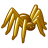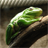# Use  Raster Property in Raster Calculater - Problem

1063
7
04-15-2014 08:35 AMby
New Contributor
Hello there,

I'm using the model builder to automate several steps.
I'm producing a raster and then I calculate the standard deviation using the tool "Get Raster Properties". After that, I want to extract all cells above the standard deviation. I do this with the "Raster Calculator" and the following expression:

Con( "%Raster%" >= float(%Property%), 1, 0 )

I always get this error: TypeError: float() takes at most 1 argument (2 given).

When I manually calculate the property value it is 2,44945784279457E-05.
My computer language is set to German, I use ArcGIS in English, can that be the problem, that the decimal value is separated by a comma and not by a point?

Would be great if you could support me,
Thanks!
Tags (3)
7 RepliesFrequent Contributor
Looking at ArcGIS help, it looks like you can't use float value in your expression in model builder.  Make sure you use a supported variable type.

http://resources.arcgis.com/en/help/main/10.1/index.html#//009z000000z7000000by
New Contributor
Hello,

The float conversion was automatically done when I double clicked the Property.

I tried now different methods, but no one doesn't work.
- If I use round up or instead of Float Int(%Property%) I get this error: TypeError: Int() takes exactly 1 argument (2 given)
- If I just put "%Property%" in it I get an error that the dataset is not supported.

Isn't it possible to calculate the standard deviation of a raster within the Model Builder and the extract all cells above this value?
I would'nt mind in which way I can do it, as long as it works. Can I do anything else so that it will work with the Model Builder?
Or can I convert the property value with another tool to a long or double?

I hope for support,
Thank you!Frequent Contributor
I'm guessing Property is the field name you are evaluating?  The problem would be the field type then, since it is listed as float.  I would see if you can change the field type to Double.by
New Contributor
Yes Property is the calculated standard deviation value which I get from the tool "Get Raster Properties".
There I just can set the type, eg. mean, standard deviation, max, min, ...

The type of the Property Field is set to Variant, I cannot change that.

Any other suggestions?
I just need to extract all values above the standard deviation - is there any other possibilty to get this value?by
New Contributor
I found out the problem, when I tried the following expressions:

Con( "%Raster%" >= Float(2,0), 1, 0 ) -> error
Con( "%Raster%" >= Float(2.0), 1, 0 ) -> worked

So it seems that the value in the float statement has to be separated by a dot and not by a comma.
As my language on the computer is set to German, I will try it tomorrow on a computer with the language English, and hopefully the calculated values are separated by a dot.

I will let you know if it worked,
If you have any other suggestions, I would be thankful 🙂by
New Contributor
For everyone who is interested. The problem was indeed that the value was separated with a , and not with a .
Changed the language to english - no problems.Frequent Contributor
Yes, that would be the problem.  I only ever saw the "Property" so I didn't realize you were using commas instead of periods.  When you use commas, that is how it separates parameters, so it thought you were giving 2 inputs instead of one.  Mark this as answered since you got it figured out.